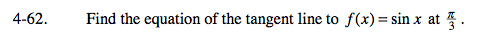### Home > CALC > Chapter Ch4 > Lesson 4.2.2 > Problem4-62

4-62.$\text{Find a point for your equation by finding }f(x) \text{ at }\frac{\pi }{3}$

$\text{To find the slope, find }f'(x)\text{ and evaluate at }\frac{\pi }{3}$

Find the equation of the tangent line by using the point-slope formula, yy1 = m(xx1),and substituting (x1, y1) and 'm' with the values and slope you found in Hint 1 and Hint 2.

$f'(x)=\text{cos}x, \ f\left ( \frac{\pi }{3} \right )=\text{sin}\frac{\sqrt{3}}{2}, \ f'\left ( \frac{\pi }{3} \right )=\text{cos}\frac{\pi }{3}= \frac{1}{2}, \ \text{ tangent line is }y-\frac{\sqrt{3}}{2}=\frac{1}{2}\left ( x-\frac{\pi }{3} \right )\text{ or }y= \frac{1}{2}x+\frac{\sqrt{3}}{2}-\frac{\pi }{6}$